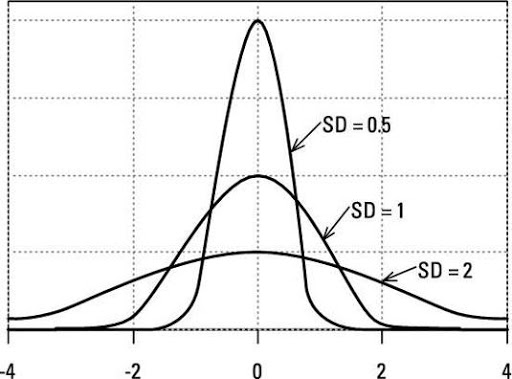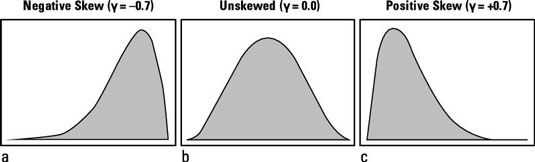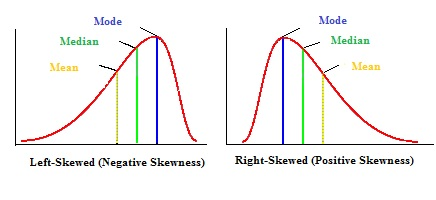# Topic 2 Measures of central tendency and dispersion

## 2.1 Central tendency

• Mean
• Median
• Mode

Example

We asked 10 people to report the number of books they each read last month.

They reported the following:

5, 3, 4, 3, 0, 1, 4, 4, 8, 0.

Some notation

Before we calculate the mode we need to know the following notation (this will also be useful for calculating the mean, variance, standard deviation…)

• $$x$$: refers to each value taken by a given variable in the sample. In this example, the variable “number of books” takes the values: 0, 1, 3, 4, 5 and 8.
• $$f$$: refers to the frequency of each of the $$x$$ values (the number of times individuals reported this value)

Easy way to calculte: write the following table:

Values in order: 0, 0, 1, 3, 3, 4, 4, 4, 5, 8.

x f fx
0 2 0
1 1 1
3 2 6
4 3 12
5 1 5
8 1 8
$$\sum x = 21$$ $$\sum f=10$$ $$\sum fx=32$$

### 2.1.1 Mean

The mean is the unweighted average of the values in the sample.

$\overline{x} = \frac{1}{n}\sum x_i$ Note tha $$x_i$$ refers to each observation reported in the sample (it is not the same as $$x$$).

This can take a long time to calculate by hand.

Easier way to calculate the mean (see Prof. Hassad’s notes):

$\overline{x} = \frac{\sum fx}{\sum f} = \frac{32}{10} = 3.2$

### 2.1.2 Median

Step 1: list all values in ascending order.

0, 0, 1, 3, 3, 4, 4, 4, 5, 8.

Step 2: Do I have an odd or even total number of reported values?

Even total: There are two middle values. The median is the average between those two values.

In the number of books example, the median is: $Median = \frac{3+4}{2} = 3.5$

Odd total: There is only one middle value. The median is this middle value.

### 2.1.3 Mode

The mode is the value with highest frequency (highest f).

x f
0 2
1 3
3 3
4 3
5 1
8 1

All of those are the mode.

Intuition: How many winners are there if runners tie in first place in a given competition?

x f
0 1
1 1
3 1
4 1
5 1
8 1

Here there is no mode.

Normal distribution:

Mean = Median = Mode

Mode, Mean, and Meadian are useful to discribe the shape of a distribution.

But they are not enough! We need new tools.

## 2.2 Measures of dispersion

• Variance
• Standard deviation
• Range
• Skewness### 2.2.1 Standard deviation

Use the same table and expand it:

Note that $$\overline{x}$$ is the mean you just calculated.

$$x$$ f fx $$x - \overline{x}$$ $$(x - \overline{x})^2$$ $$f(x - \overline{x})^2$$
. $$\sum f$$ $$\sum fx$$ $$\sum above = A$$

Calculate the standard deviation:

Or, more formally:

$sd = \sqrt{\frac{f(x - \overline{x})^2}{n-1} }$

$sd = \sqrt{\frac{A}{n-1} }$

Note that the standard deviation of a standard normal distribution is 1.

### 2.2.2 Variance:

$variance = sd^2$

Note that the variance of a standard normal distribution is 1.

Range = Max-Min

### 2.2.4 Skewness

• If skewness is more than 1 or less than -1, then we have a highly skewed distribution.
• If skewness is between -1 and -1/2 or between 1 and 1/2, then we have a moderatly skewed distribution.
• If skewness is between -1/2 and 1/2, then we have an approximatly symmetric distribution (or a negligible degree of skewness).### 2.2.5 Example of calculations

The following are data for the variable: number of movies watched in the past month.

1; 5; 5; 4; 5; 4; 0; 15; 1; 0; 0; 1.

Calculate: mean, media, mode, variance, standard deviation and range.

$$x$$ f fx $$x - \overline{x}$$ $$(x - \overline{x})^2$$ $$f(x - \overline{x})^2$$
0 3 0 -3.4 11.56 34.68
1 3 3 -2.4 5.76 17.28
4 2 8 0.6 0.36 0.72
5 3 15 1.6 2.56 7.68
15 1 15 11.6 134.56 134.56
. $$\sum f = 12$$ $$\sum fx = 41$$ $$\sum above = 194.92$$

$\overline{x} = \frac{\sum fx}{\sum f} = \frac{41}{12} = 3.4$

Mode = 0 , 1 , 5

Median = (4 + 1) / 2 = 2.5

$sd = \sqrt{\frac{194.92}{11} } = \sqrt{17.72} = 4.2$ $sd^2 = 17.72$

$Range = 15 - 0 = -15$